# Number Power

The Number Power books employ traditional math instruction to target particular sets of math skills. They contain straightforward explanations; easy-to-follow, step-by-step instructions featuring real-life examples; and extensive reinforcement exercises. The Exam View CD lets instructors create printable worksheets and tests for students based on the skills for which they need additional practice or assessment.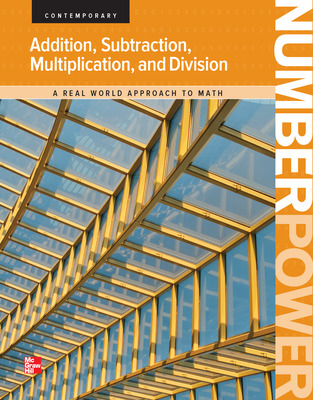The Number Power books use traditional math instruction to target particular sets of math skills. Topics range from addition, subtraction, multiplication, and division to algebra to financial literacy. All topics are presented with straightforward explanations; easy-to-follow, step-by-step instructions featuring real-life examples; and extensive reinforcement exercises help ensure mastery. The Exam View CD lets instructors create printable worksheets and tests for students based on the skills for which they need additional practice or assessment.

Features include:

• Short, manageable lessons and exercises
• Step-by-step examples and relevant visuals
• Extensive exercises for skills practice
• Regular skill inventories and reviews
• Diagnostic pre-tests and evaluative post-tests
• Performance-based prescriptions for additional study
• Glossary of math terms
• Self-assessment chart

## Program Components

•Number Power: Addition, Subtraction, Multiplication, and Division, Student Edition
•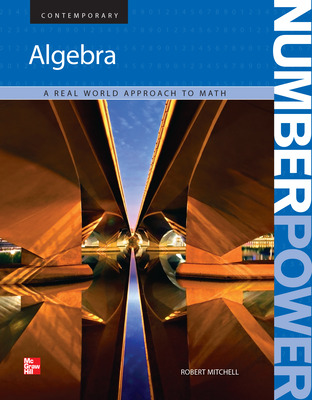Number Power: Algebra, Student Edition
•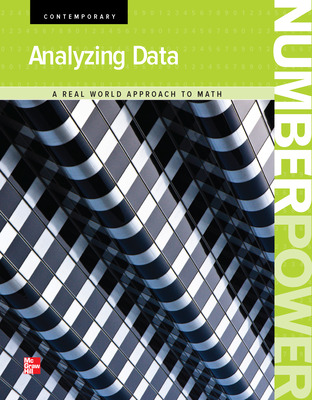Number Power: Analyzing Data, Student Edition
•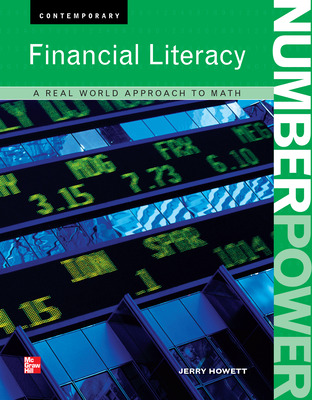Number Power: Financial Literacy
•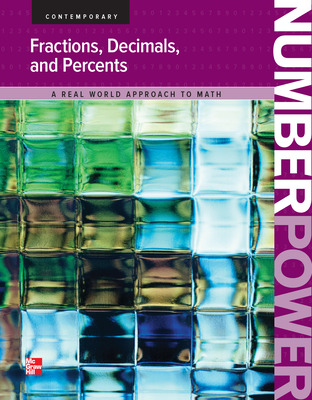Number Power: Fractions, Decimals, and Percents, Student Edition
•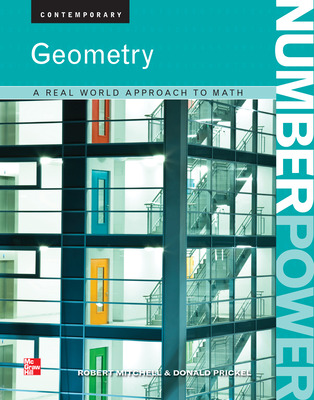Number Power: Geometry, Student Edition
•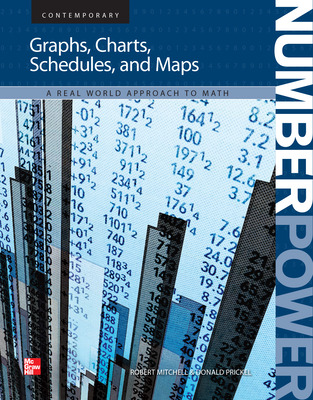Number Power: Graphs, Tables, Schedules, and Maps, Student Edition
•Number Power: Measurement, Student Edition
•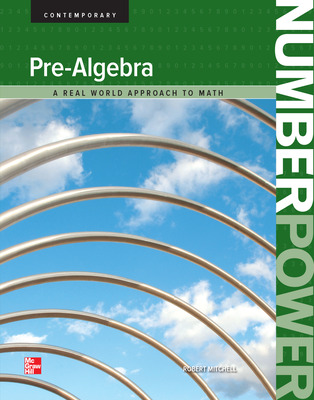Number Power: Pre-Algebra, Student Edition
•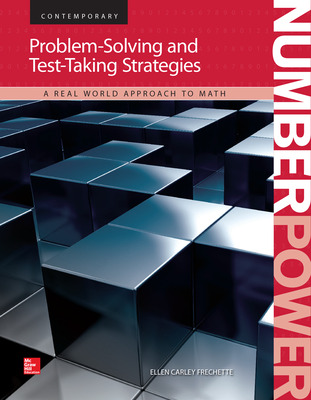Number Power: Problem-Solving and Test-Taking Strategies, Student Edition
•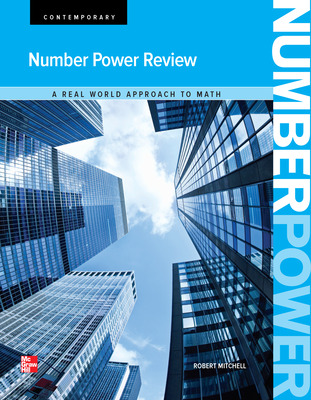Number Power: Review
•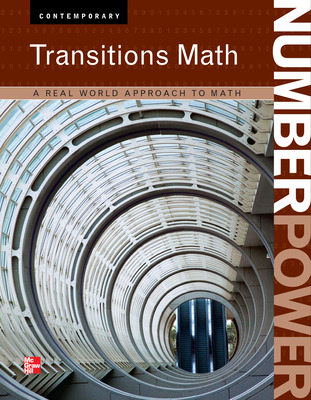Number Power: Transition Math
Sort by: